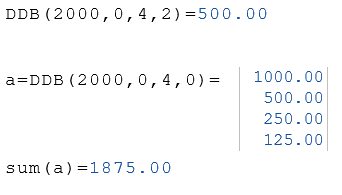# DDB Function

Calculate the depreciation of an asset

## Description

The function DDB calculates a value specifying the depreciation of an asset for a specific time period using the double-declining balance method or some other method you specify.

### Syntax

DDB (Cost, Salvage, Life, Period)

DDB (Cost, Salvage, Life, Period, Factor)

### Required Parameter

Cost

The initial cost of the asset.

Salvage

The value of the asset at the end of its useful life.

Life

The length of useful life of the asset.

Period

The period for which asset depreciation is calculated.
New from version 7.10:
If a zero is specified as Period, the result is a list containing the values of all periods.

### Optionaler Parameter

Factor

The rate at which the balance declines. If omitted, 2 (double-declining method) is assumed.

### Examples